# Can we use B-splines to generate non-linear data?

October 15, 2017
By

Want to share your content on R-bloggers? click here if you have a blog, or here if you don't.

I’m exploring the idea of adding a function or set of functions to the `simstudy` package that would make it possible to easily generate non-linear data. One way to do this would be using B-splines. Typically, one uses splines to fit a curve to data, but I thought it might be useful to switch things around a bit to use the underlying splines to generate data. This would facilitate exploring models where we know the assumption of linearity is violated. It would also make it easy to explore spline methods, because as with any other simulated data set, we would know the underlying data generating process.

### B-splines

A B-spline is a linear combination of a set of basis functions that are determined by the number and location of specified knots or cut-points, as well as the (polynomial) degree of curvature. A degree of one implies a set of straight lines, degree of two implies a quadratic curve, three a cubic curve, etc. This nice quick intro provides much more insight into issues B-splines than I can provide here. Or if you want even more detail, check out this book. It is a very rich topic.

Within a cut-point region, the sum of the basis functions always equals 1. This is easy to see by looking at a plot of basis functions, several of which are provided below. The definition and shape of the basis functions do not in any way depend on the data, only on the degree and cut-points. Of course, these functions can be added together in infinitely different ways using weights. If one is trying to fit a B-spline line to data, those weights can be estimated using regression models.

## Splines in R

The `bs` function in the `splines` package, returns values from these basis functions based on the specification of knots and degree of curvature. I wrote a wrapper function that uses the `bs` function to generate the basis function, and then I do a linear transformation of these functions by multiplying the vector parameter theta, which is just a vector of coefficients. The linear combination at each value of \(x\) (the support of the basis functions) generates a value (which I call \(y.spline\)) on the desired curve. The wrapper returns a list of objects, including a data.table that includes \(x\) and \(y.spline\), as well as the basis functions, and knots.

``````library(splines)
library(data.table)
library(ggplot2)
library(broom)

genSpline <- function(x, knots, degree, theta) {

basis <- bs(x = x, knots = knots, degree = degree,
Boundary.knots = c(0,1), intercept = TRUE)

y.spline <- basis %*% theta

dt <- data.table(x, y.spline = as.vector(y.spline))

return(list(dt = dt, basis = basis, knots = knots))

}``````

I’ve also written two functions that make it easy to print the basis function and the spline curve. This will enable us to look at a variety of splines.

``````plot.basis <- function(basisdata) {

dtbasis <- as.data.table(basisdata\$basis)
dtbasis[, x := seq(0, 1, length.out = .N)]
dtmelt <- melt(data = dtbasis, id = "x",
variable.name = "basis", variable.factor = TRUE)

ggplot(data=dtmelt, aes(x=x, y=value, group = basis)) +
geom_line(aes(color=basis), size = 1) +
theme(legend.position = "none") +
scale_x_continuous(limits = c(0, 1),
breaks = c(0, basisdata\$knots, 1)) +
theme(panel.grid.minor = element_blank())
}``````
``````plot.spline <- function(basisdata, points = FALSE) {

p <- ggplot(data = basisdata\$dt)

if (points) p <- p + geom_point(aes(x=x, y = y), color = "grey75")

p <- p +
geom_line(aes(x = x, y = y.spline), color = "red", size = 1) +
scale_y_continuous(limits = c(0, 1)) +
scale_x_continuous(limits = c(0, 1), breaks = knots) +
theme(panel.grid.minor = element_blank())

return(p)

}``````

### Linear spline with quartile cut-points

Here is a simple linear spline that has four regions defined by three cut-points, and the slope of the line in each region varies. The first value of theta is essentially the intercept. When you look at the basis plot, you will see that any single region has two “active” basis functions (represented by two colors), the other functions are all 0 in that region. The slope of the line in each is determined by the relevant values of theta. It is probably just easier to take a look:

``````x <- seq(0, 1, length.out = 1000)

knots <- c(0.25, 0.5, 0.75)
theta = c(0.6, 0.1, 0.3, 0.2, 0.9)

sdata <- genSpline(x, knots, 1, theta)``````

For this example, I am printing out the basis function for the first few values of \(x\).

``round( head(cbind(x = sdata\$dt\$x, sdata\$basis)), 4 )``
``````##          x     1     2 3 4 5
## [1,] 0.000 1.000 0.000 0 0 0
## [2,] 0.001 0.996 0.004 0 0 0
## [3,] 0.002 0.992 0.008 0 0 0
## [4,] 0.003 0.988 0.012 0 0 0
## [5,] 0.004 0.984 0.016 0 0 0
## [6,] 0.005 0.980 0.020 0 0 0``````
``plot.basis(sdata)``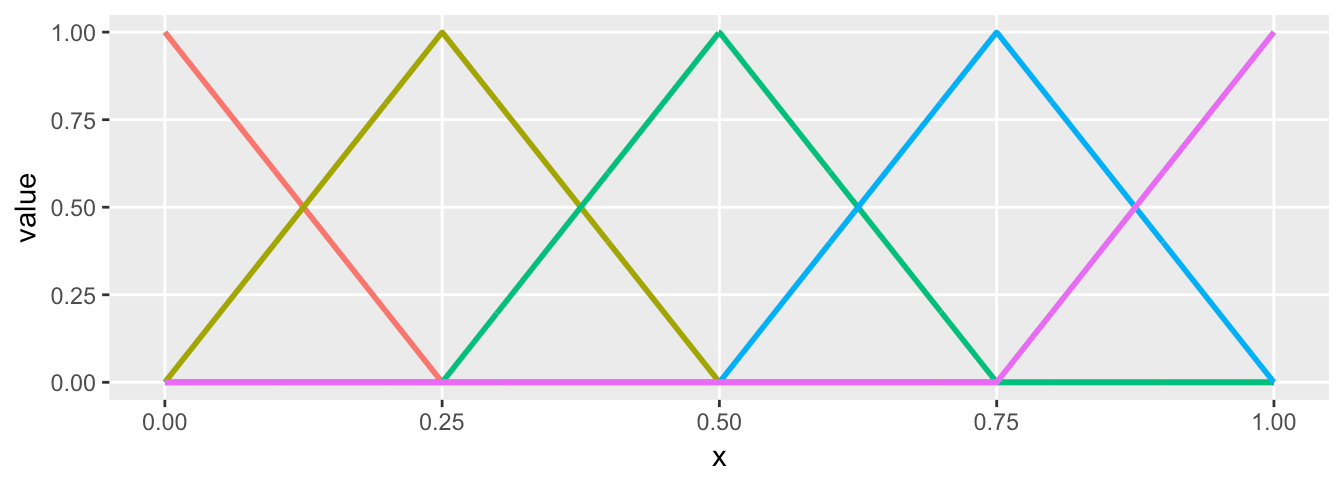``plot.spline(sdata)``### Same knots (cut-points) but different theta (coefficients)

If use the same knot and degree specification, but change the vector \(theta\), we change the slope of the lines in each of the four regions:

``````theta = c(0.2, 0.3, 0.8, 0.2, 0.1)
sdata <- genSpline(x, knots, 1, theta)

plot.basis(sdata)````````plot.spline(sdata)``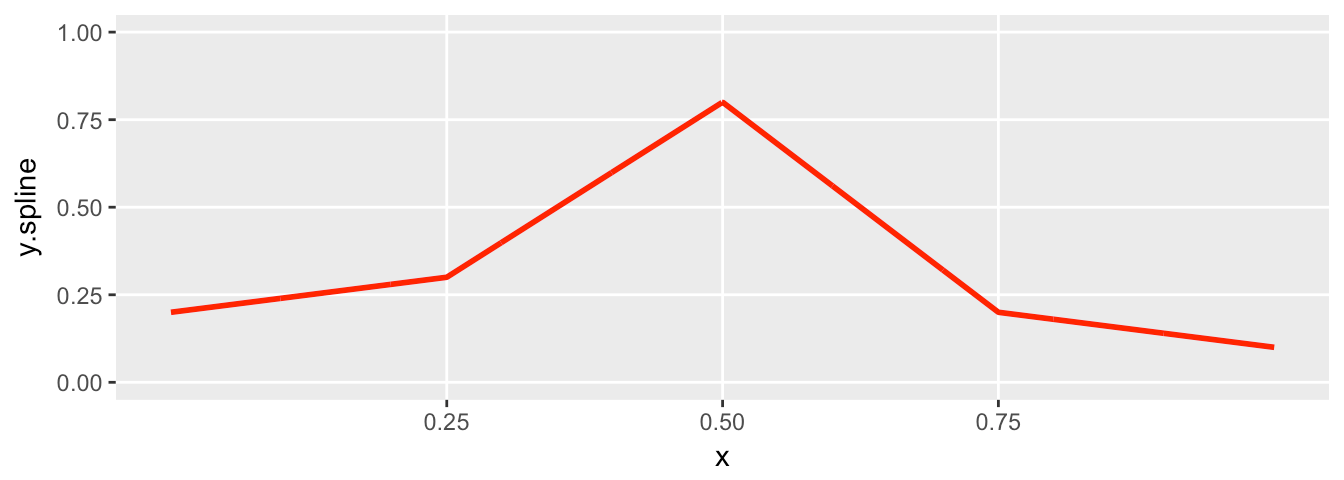### Quadratic spline with quartile cut-points

The basis functions get a little more elaborate with a quadratic spline. With this added degree, we get an additional basis function in each region, so you should see 3 colors instead of 2. The resulting spline is parabolic in each region, but with a different shape, each of which is determined by theta.

``````knots <- c(0.25, 0.5, 0.75)
theta = c(0.6, 0.1, 0.5, 0.2, 0.8, 0.3)

sdata <- genSpline(x, knots, 2, theta)

plot.basis(sdata)````````plot.spline(sdata)``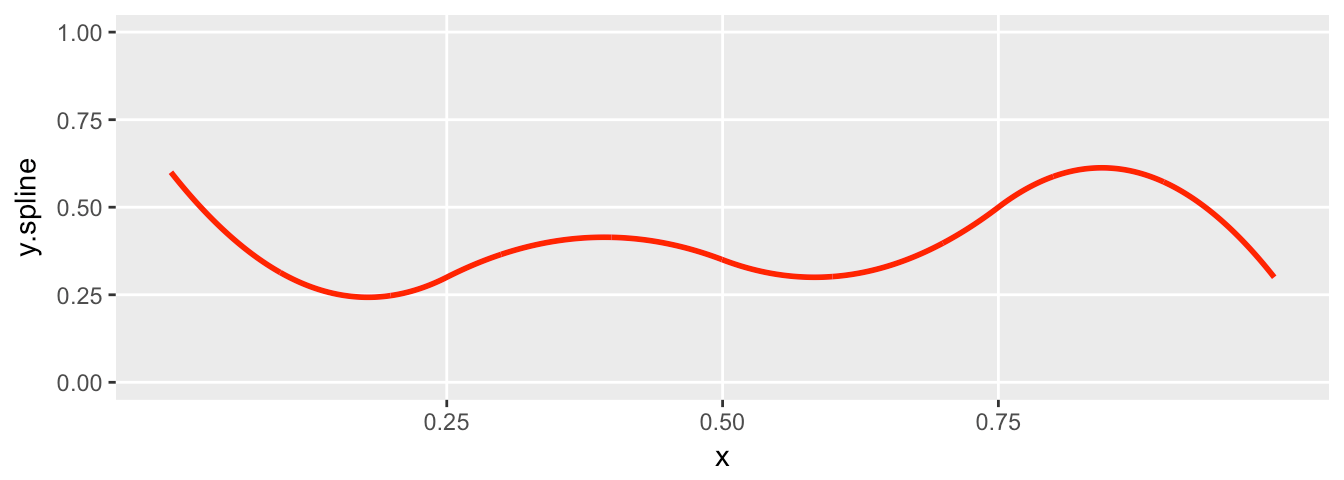### Quadratic spline with two cut-points (three regions)

``````knots <- c(0.333, 0.666)
theta = c(0.2, 0.4, 0.1, 0.9, 0.6)

sdata <- genSpline(x, knots, 2, theta)
plot.basis(sdata)``````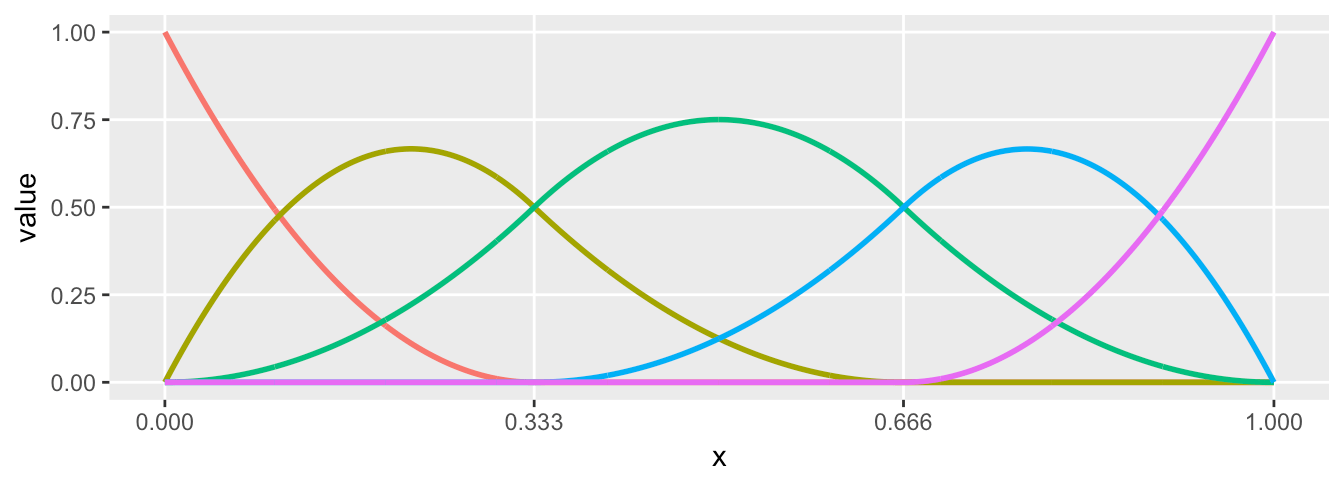``plot.spline(sdata)``### Cubic spline with two cut-points (three regions)

And in this last example, we generate basis functions for a cubic spline the differs in three regions. The added curvature is apparent:

``````knots <- c(0.333, 0.666)
theta = c(0.2, 0.6, 0.1, 0.9, 0.2, 0.8)

sdata <- genSpline(x, knots, 3, theta)
plot.basis(sdata)``````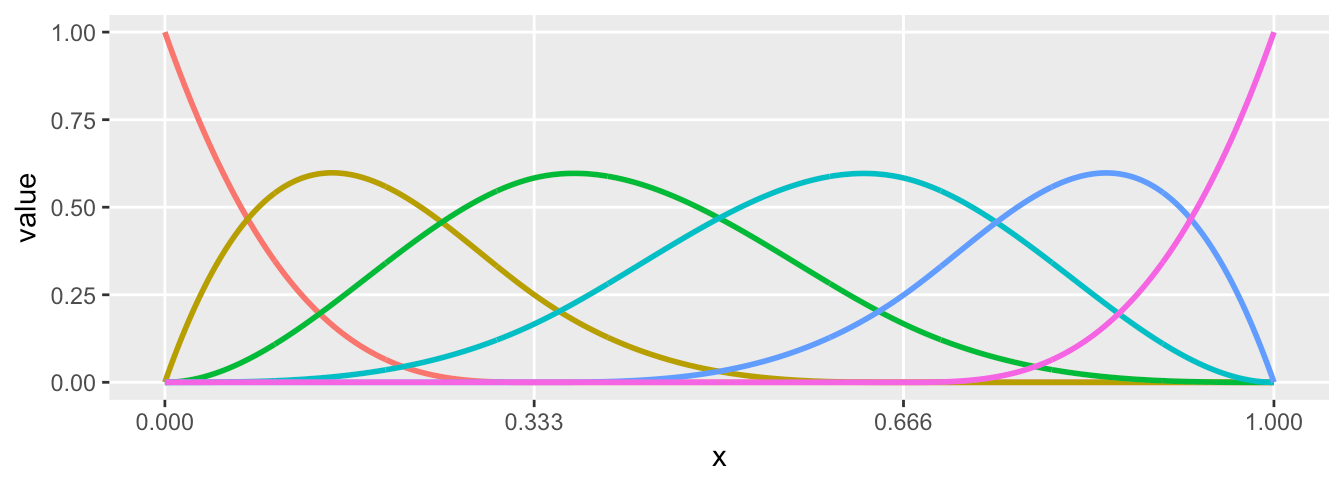``plot.spline(sdata)``### Generating new data from the underlying spline

It is a simple step to generate data from the spline. Each value on the line is treated as the mean, and “observed” data can be generated by adding variation. In this case, I use the normal distribution, but there is no reason other distributions can’t be used. I’m generating data based on the the parameters in the previous example. And this time, the spline plot includes the randomly generated data:

``````set.seed(5)
x <- runif(250)
sdata <- genSpline(x, knots, 3, theta)

sdata\$dt[,  y := rnorm(.N, y.spline, 0.1)]

plot.spline(sdata, points = TRUE)``````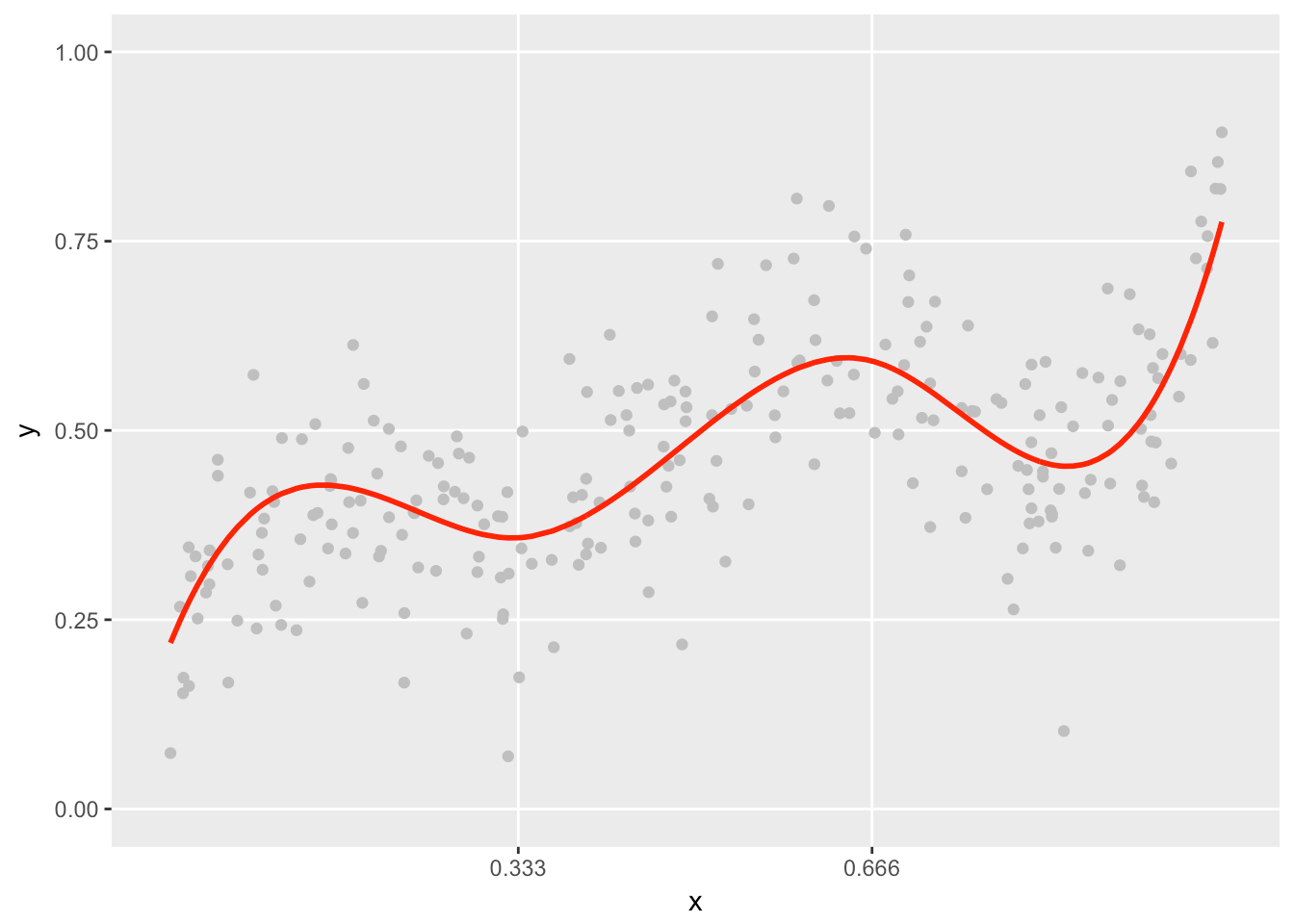Now that we have generated new data, why don’t we go ahead and fit a model to see if we can recover the coefficients specified in theta? We are interested in the relationship of \(x\) and \(y\), but the relationship is not linear and changes across \(x\). To estimate a model, we regress the outcome data \(y\) on the values of the basis function that correspond to each value of \(x\):

``````dxbasis <- as.data.table(sdata\$basis)
setnames(dxbasis, paste0("x", names(dxbasis)))

dxbasis[, y := sdata\$dt\$y]

round(dxbasis, 3)``````
``````##         x1    x2    x3    x4    x5    x6     y
##   1: 0.063 0.557 0.343 0.036 0.000 0.000 0.443
##   2: 0.000 0.000 0.140 0.565 0.295 0.000 0.542
##   3: 0.000 0.000 0.003 0.079 0.495 0.424 0.634
##   4: 0.003 0.370 0.523 0.104 0.000 0.000 0.232
##   5: 0.322 0.553 0.120 0.005 0.000 0.000 0.269
##  ---
## 246: 0.000 0.023 0.442 0.494 0.041 0.000 0.520
## 247: 0.613 0.356 0.031 0.001 0.000 0.000 0.440
## 248: 0.246 0.584 0.161 0.009 0.000 0.000 0.236
## 249: 0.000 0.000 0.014 0.207 0.597 0.182 0.505
## 250: 0.002 0.344 0.539 0.115 0.000 0.000 0.313``````
``````# fit the model - explicitly exclude intercept since x1 is intercept

lmfit <- lm(y ~ x1 + x2 + x3 + x4 + x5 + x6 - 1, data = dxbasis)
cbind(tidy(lmfit)[,1:3], true = theta)``````
``````##   term   estimate  std.error true
## 1   x1 0.16465186 0.03619581  0.2
## 2   x2 0.57855125 0.03996219  0.6
## 3   x3 0.09093425 0.04267027  0.1
## 4   x4 0.94938718 0.04395370  0.9
## 5   x5 0.13579559 0.03805510  0.2
## 6   x6 0.85867619 0.03346704  0.8``````

Using the parameter estimates (estimated here using OLS), we can get predicted values and plot them to see how well we did:

``````# get the predicted values so we can plot

dxbasis[ , y.pred := predict(object = lmfit)]
dxbasis[ , x := x]

# blue line represents predicted values

plot.spline(sdata, points = TRUE) +
geom_line(data=dxbasis, aes(x=x, y=y.pred), color = "blue", size = 1 )``````The model did quite a good job, because we happened to assume the correct underlying assumptions of the spline. However, let’s say we suspected that the data were generated by a quadratic spline. We need to get the basis function assuming the same cut-points for the knots but now using a degree equal to two. Since a reduction in curvature reduces the number of basis functions by one, the linear model changes slightly. (Note that this model is not quite nested in the previous (cubic) model, because the values of the basis functions are different.)

``````xdata <- genSpline(x, knots, 2, theta = rep(1,5))
dxbasis <- as.data.table(xdata\$basis)
setnames(dxbasis, paste0("x", names(dxbasis)))

dxbasis[, y := sdata\$dt\$y]

lmfit <- lm(y ~ x1 + x2 + x3 + x4 + x5 - 1, data = dxbasis)

dxbasis[ , y.pred := predict(object = lmfit)]
dxbasis[ , x := x]

plot.spline(sdata, points = TRUE) +
geom_line(data=dxbasis, aes(x=x, y=y.pred),
color = "forestgreen", size = 1 )``````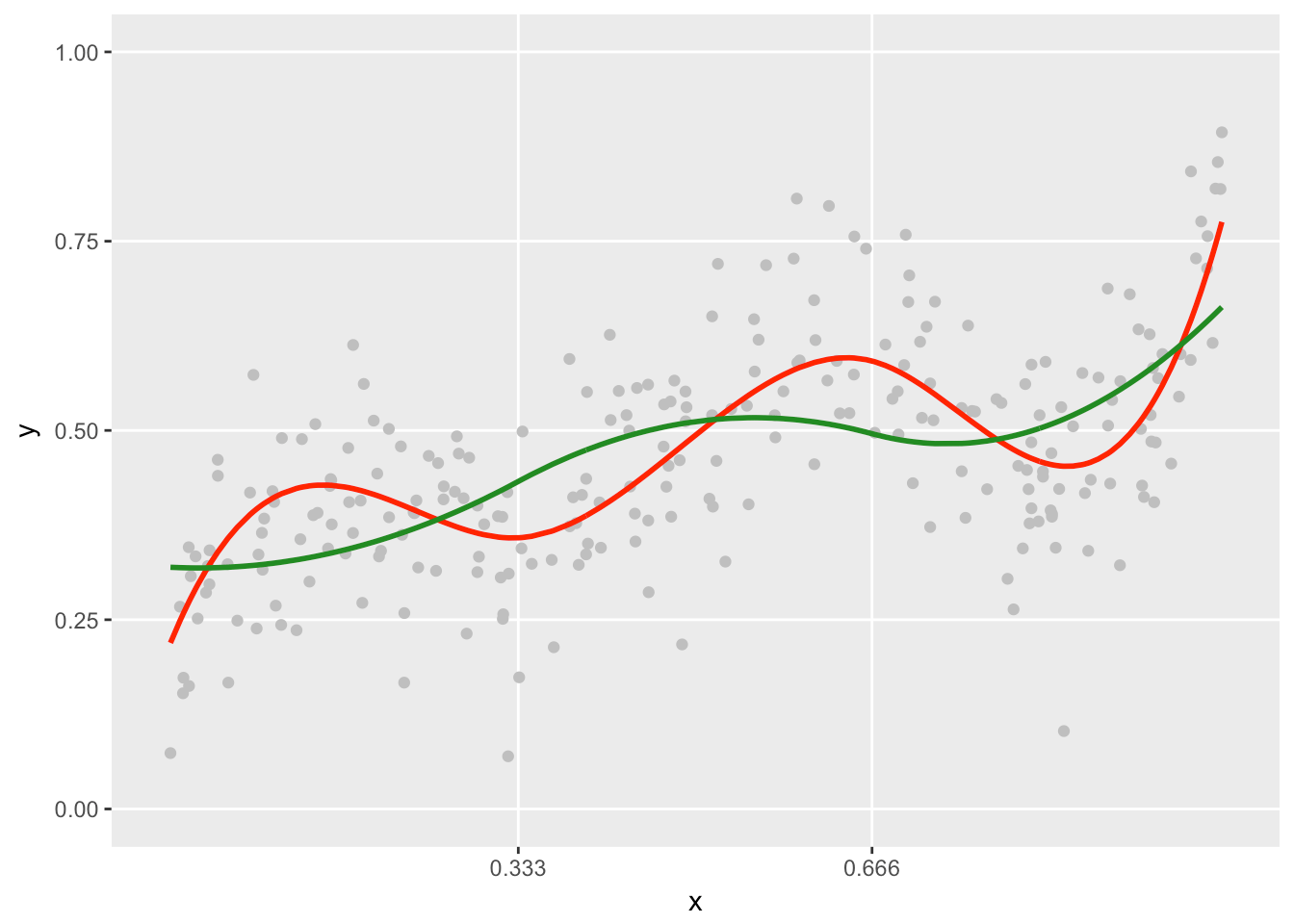If we compare the two models in terms of model fit, the cubic model only does slightly better in term of \(R^2\): 0.96 vs. 0.94. In this case, it probably wouldn’t be so obvious which model to use.

R-bloggers.com offers daily e-mail updates about R news and tutorials about learning R and many other topics. Click here if you're looking to post or find an R/data-science job.
Want to share your content on R-bloggers? click here if you have a blog, or here if you don't.